# Chpater 10 POWERPOINT JEOPARDY Lateral Area Surface Area

• Slides: 27Chpater 10!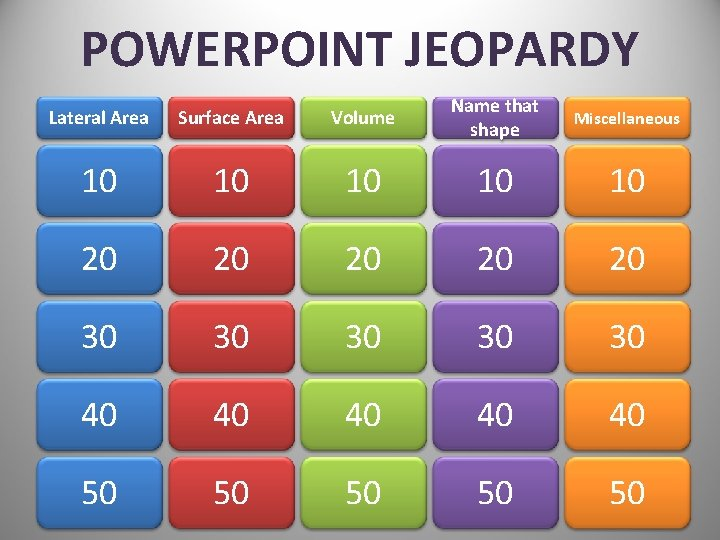POWERPOINT JEOPARDY Lateral Area Surface Area Volume Name that shape Miscellaneous 10 10 10 20 20 20 30 30 30 40 40 40 50 50 50Find the Lateral Area of the shape below.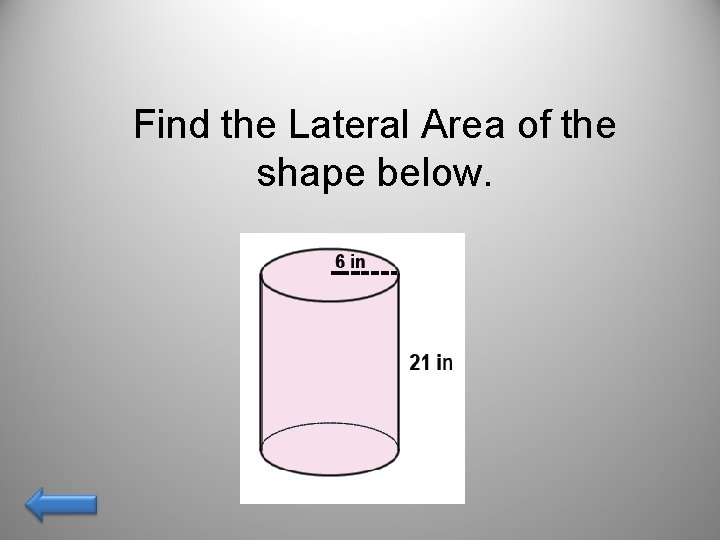Find the Lateral Area of the shape below.Find the Lateral Area of the shape below.Find the Lateral Area of the shape below.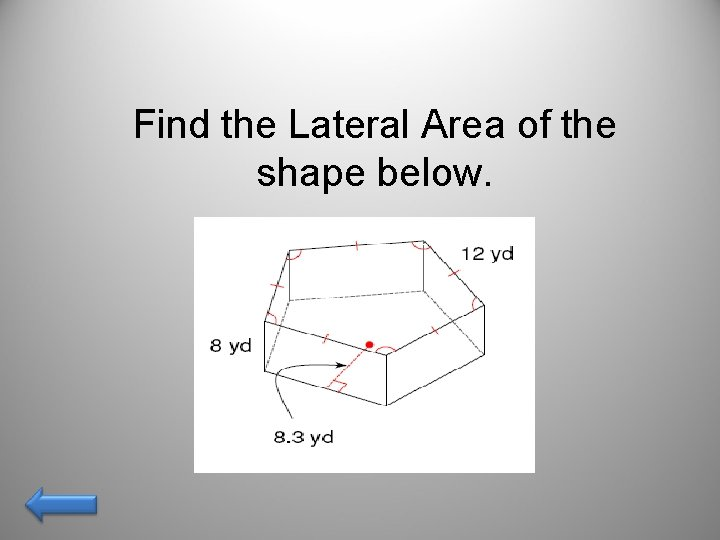Find the Lateral Area of the shape below.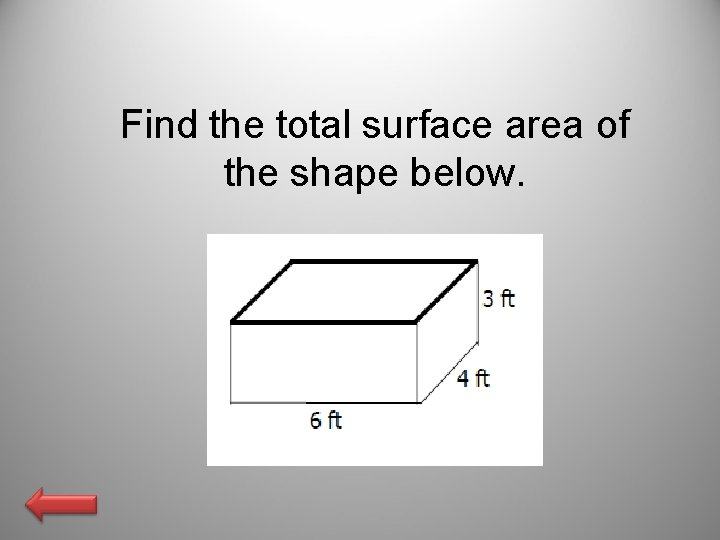Find the total surface area of the shape below.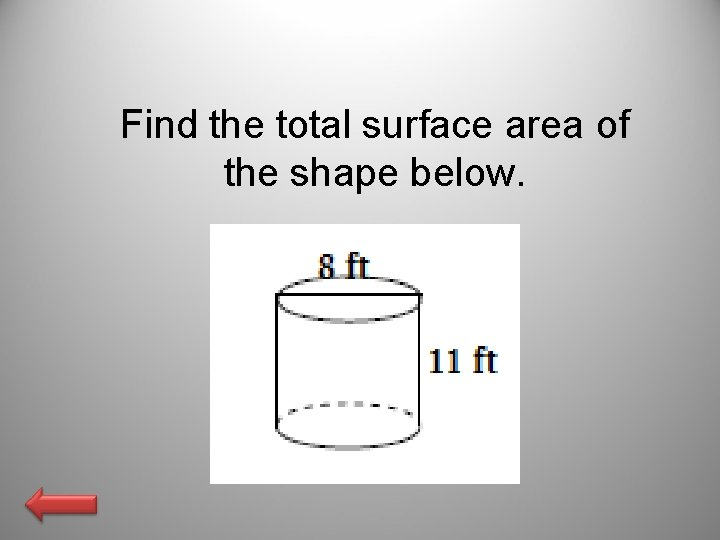Find the total surface area of the shape below.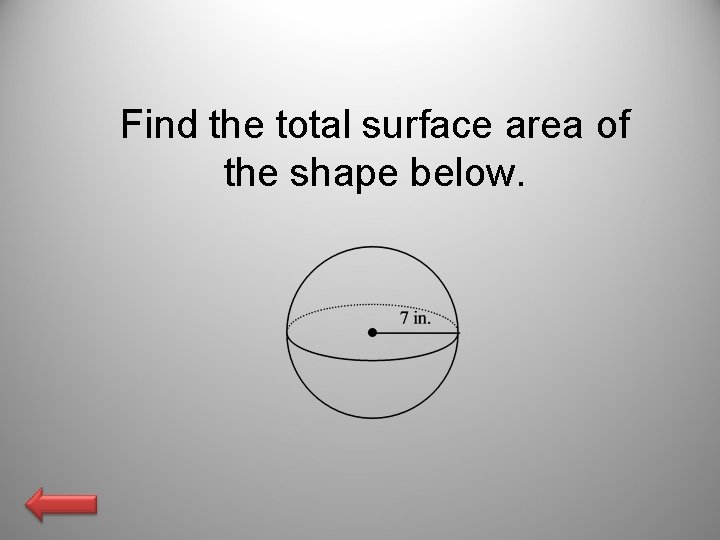Find the total surface area of the shape below.Find the surface area of a regular square pyramid with base edge length 16 m and slant height 10 m.Find the volume of the rectangular prism.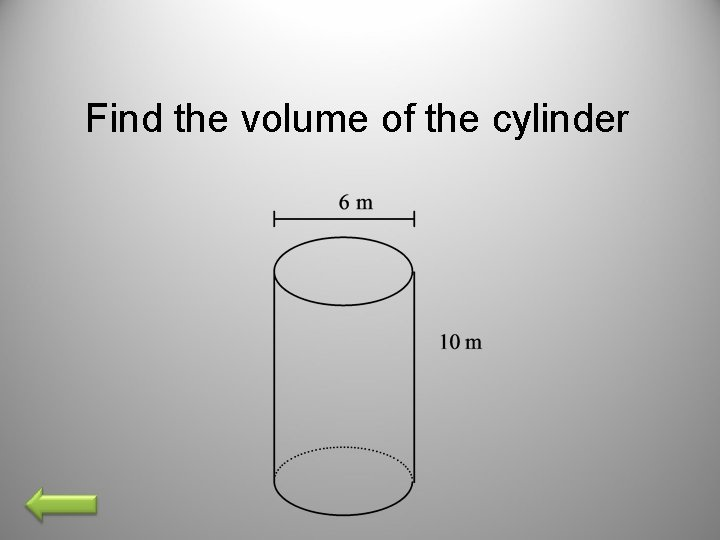Find the volume of the cylinder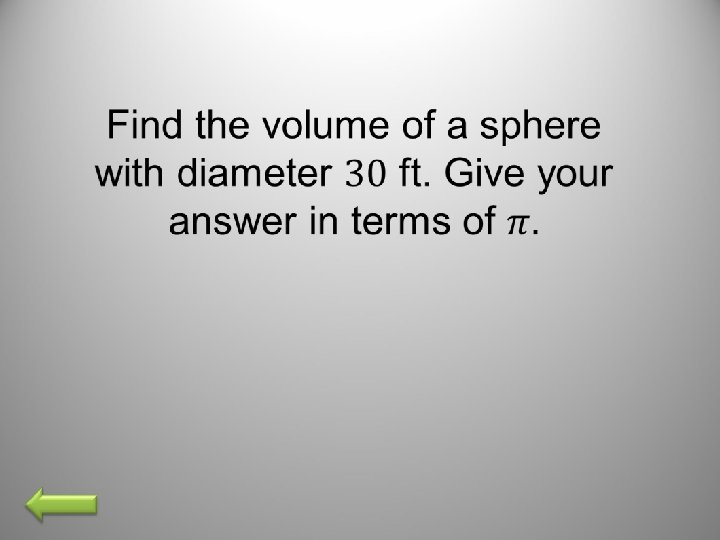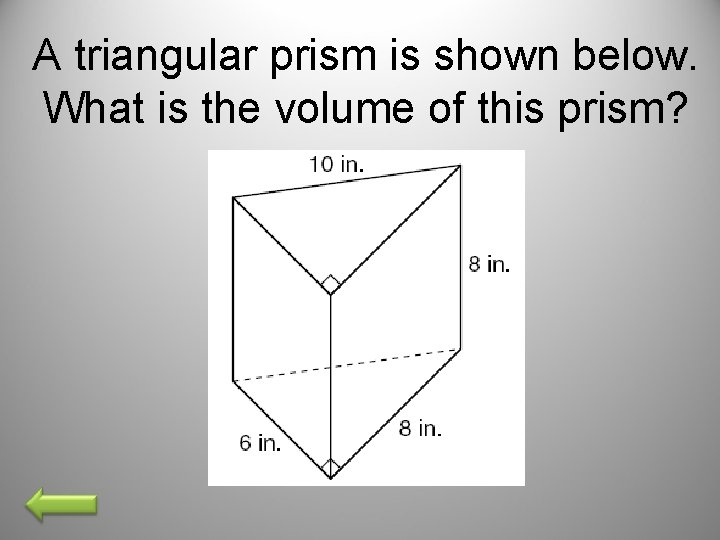A triangular prism is shown below. What is the volume of this prism?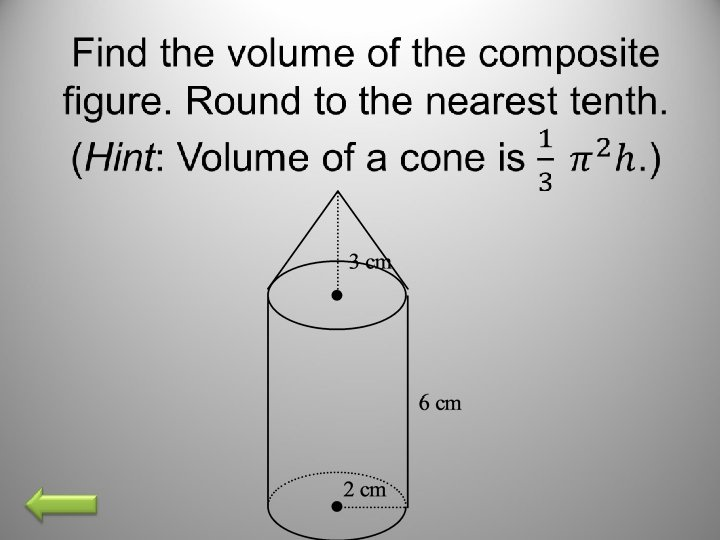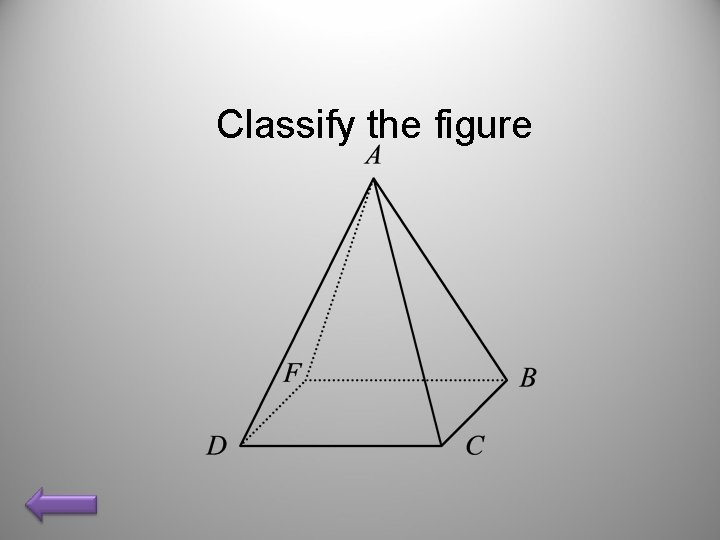Classify the figureDescribe three-dimensional figure that can be made from the given net.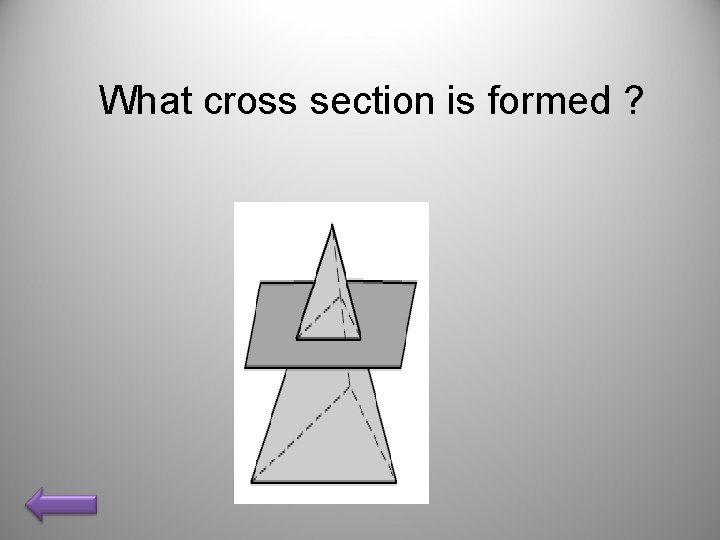What cross section is formed ?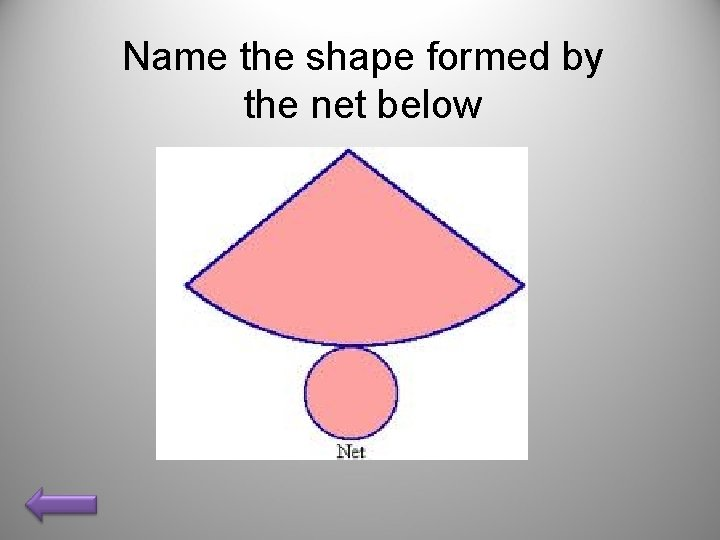Name the shape formed by the net below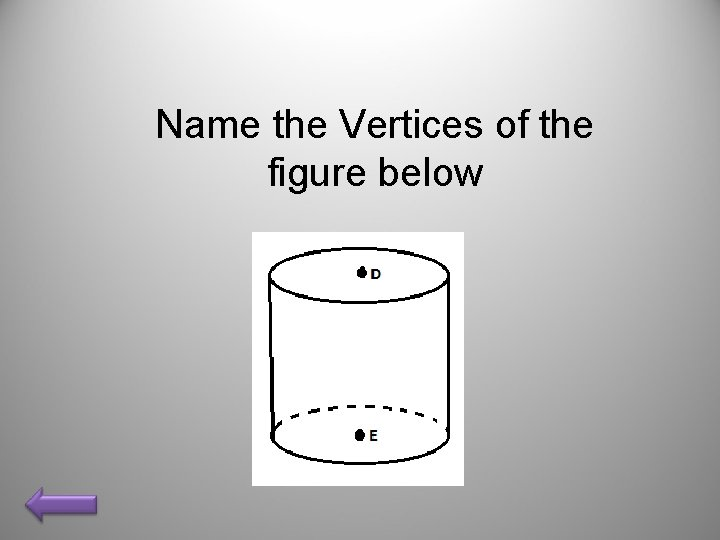Name the Vertices of the figure below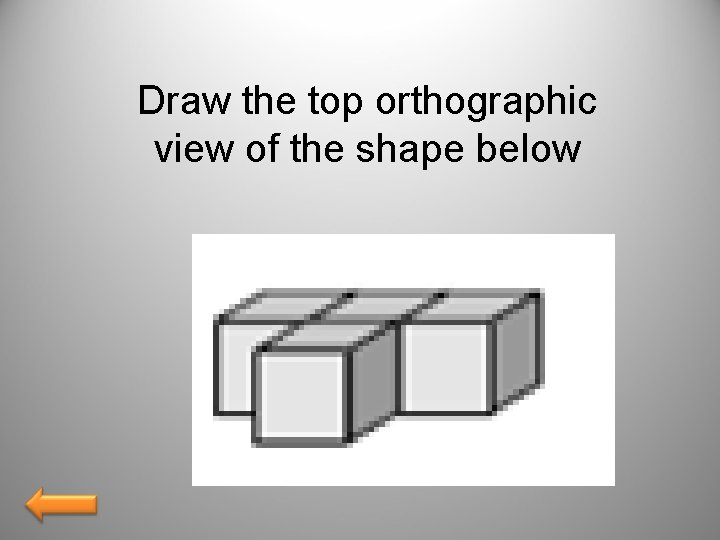Draw the top orthographic view of the shape belowIdentify the cross section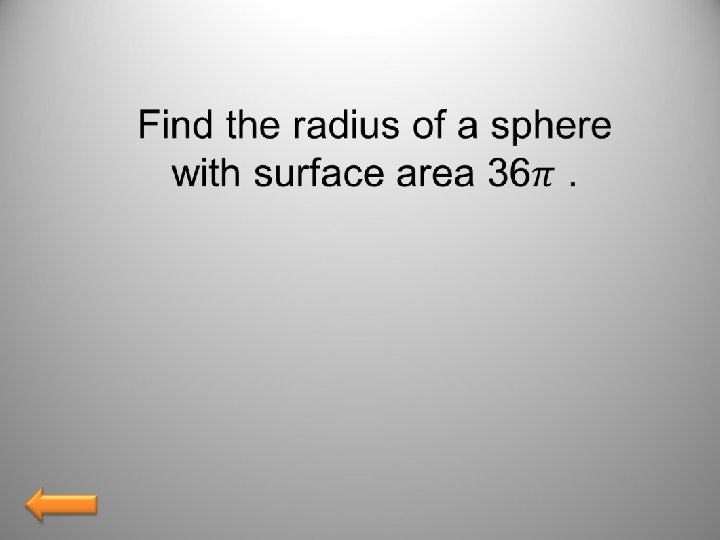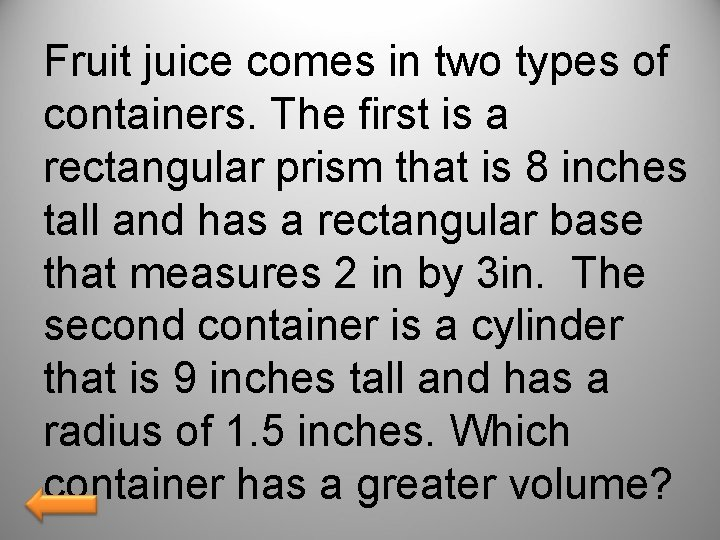Fruit juice comes in two types of containers. The first is a rectangular prism that is 8 inches tall and has a rectangular base that measures 2 in by 3 in. The second container is a cylinder that is 9 inches tall and has a radius of 1. 5 inches. Which container has a greater volume?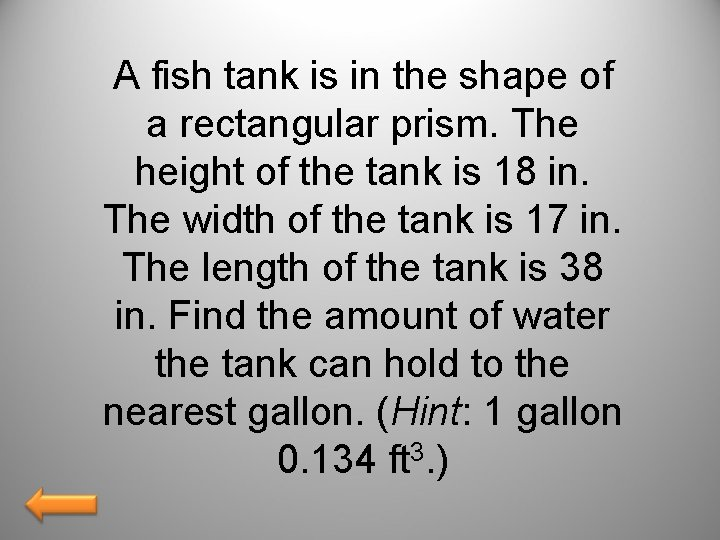A fish tank is in the shape of a rectangular prism. The height of the tank is 18 in. The width of the tank is 17 in. The length of the tank is 38 in. Find the amount of water the tank can hold to the nearest gallon. (Hint: 1 gallon 0. 134 ft 3. )